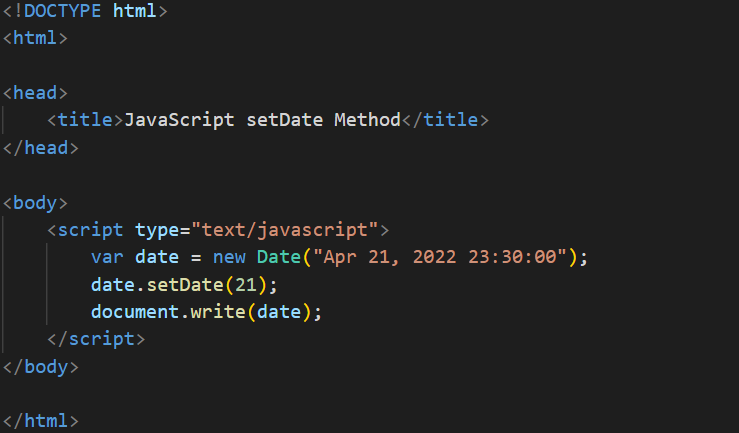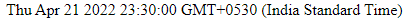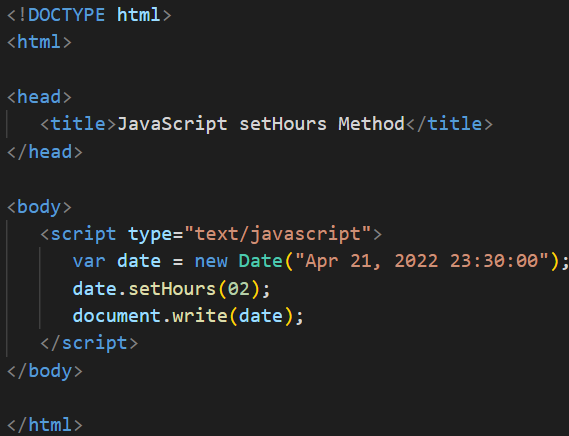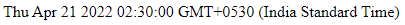JavaScript Set Date Methods

# JavaScript Set Date Methods

JavaScript Set Date Methods
Commonly used Date and Time Methods are as follows:
 Name Description setDate() Set the day of the month as an integer from 1 to 31 setHours() set the hours as an integer between 0 and 23 setMinutes() set the minutes is an integer between 0 and 59 setMonth() set the Months as an integer between 0 and 11 where 0 is January and 11 is December setSeconds() set the seconds as an integer between 0 and 59 setTime() set the current time in milliseconds where is milliseconds since January 1, 1970 setFullYear() Set the year (optionally month and day) setMilliseconds() Set the milliseconds is an integer between 0 to 999 setTime() Set the current time in milliseconds where is milliseconds since January 1, 1970
Example1OutputExample2Output